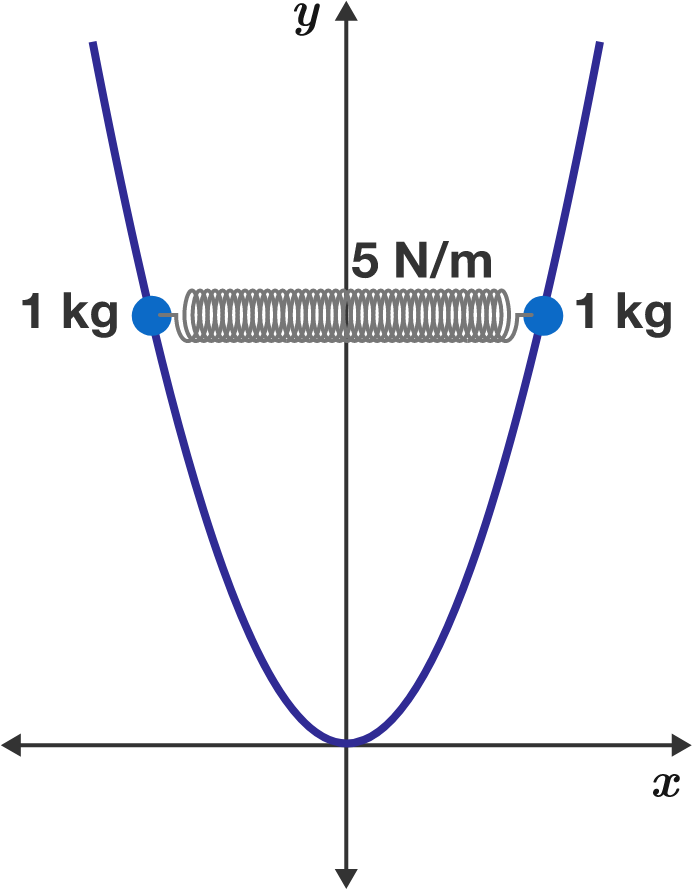# Spring Compressed on ParabolaTwo $\SI{1}{\kilo\gram}$ beads are at the ends of a compressed spring resting on a smooth parabolic wire $\big(y = x^2\big).$ The spring has a natural length of $\SI{1}{\meter}$ and a force constant of $\SI[per-mode=symbol]{5}{\newton\per\meter}.$

If the system is in static equilibrium, how far away from the $y$-axis is each bead (in meters)?

Note: The spring is horizontal and gravity is $\SI[per-mode=symbol]{10}{\meter\per\second\squared}$ in the $-y$ direction.

×